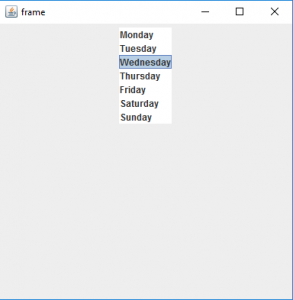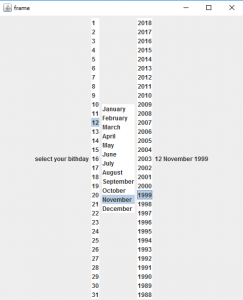Open In App

# Java Swing | JList with examples

JList is part of Java Swing package . JList is a component that displays a set of Objects and allows the user to select one or more items . JList inherits JComponent class. JList is a easy way to display an array of Vectors .
Constructor for JList are :

1. JList(): creates an empty blank list
2. JList(E [ ] l) : creates an new list with the elements of the array.
3. JList(ListModel d): creates a new list with the specified List Model
4. JList(Vector l) : creates a new list with the elements of the vector

Commonly used methods are :

The Following programs will illustrate the use of JLists
1. Program to create a simple JList

## Java

 `// java Program to create a simple JList``import` `java.awt.event.*;``import` `java.awt.*;``import` `javax.swing.*;``class` `solve ``extends` `JFrame``{``    ` `    ``//frame``    ``static` `JFrame f;``    ` `    ``//lists``    ``static` `JList b;`` `  `    ``//main class``    ``public` `static` `void` `main(String[] args)``    ``{``        ``//create a new frame``        ``f = ``new` `JFrame(``"frame"``);``        ` `        ``//create a object``        ``solve s=``new` `solve();``      ` `        ``//create a panel``        ``JPanel p =``new` `JPanel();``        ` `        ``//create a new label``        ``JLabel l= ``new` `JLabel(``"select the day of the week"``);` `        ``//String array to store weekdays``        ``String week[]= { ``"Monday"``,``"Tuesday"``,``"Wednesday"``,``                         ``"Thursday"``,``"Friday"``,``"Saturday"``,``"Sunday"``};``        ` `        ``//create list``        ``b= ``new` `JList(week);``        ` `        ``//set a selected index``        ``b.setSelectedIndex(``2``);``        ` `        ``//add list to panel``        ``p.add(b);`` ` `        ``f.add(p);``        ` `        ``//set the size of frame``        ``f.setSize(``400``,``400``);``         ` `        ``f.show();``    ``}``    ` `    ` `}`

Output :2. Program to create a list and add itemListener to it (program to select your birthday using lists) .

## Java

 `// java Program to create a list and add itemListener to it``// (program to select your birthday using lists) .``import` `javax.swing.event.*;``import` `java.awt.*;``import` `javax.swing.*;``class` `solve ``extends` `JFrame ``implements` `ListSelectionListener``{``    ` `    ``//frame``    ``static` `JFrame f;``    ` `    ``//lists``    ``static` `JList b,b1,b2;``    ` `    ``//label``    ``static` `JLabel l1;`` `  `    ``//main class``    ``public` `static` `void` `main(String[] args)``    ``{``        ``//create a new frame``        ``f = ``new` `JFrame(``"frame"``);``        ` `        ``//create a object``        ``solve s=``new` `solve();``      ` `        ``//create a panel``        ``JPanel p =``new` `JPanel();``        ` `        ``//create a new label``        ``JLabel l= ``new` `JLabel(``"select your birthday"``);``        ``l1= ``new` `JLabel();` `        ``//String array to store weekdays``        ``String month[]= { ``"January"``, ``"February"``, ``"March"``,``        ``"April"``, ``"May"``, ``"June"``, ``"July"``, ``"August"``,``        ``"September"``, ``"October"``, ``"November"``, ``"December"``};``        ` `        ``//create a array for months and year``        ``String date[]=``new` `String[``31``],year[]=``new` `String[``31``];``        ` `        ``//add month number and year to list``        ``for``(``int` `i=``0``;i<``31``;i++)``        ``{``            ``date[i]=``""``+(``int``)(i+``1``);``            ``year[i]=``""``+(``int``)(``2018``-i);``        ``}``        ` `        ``//create lists``        ``b= ``new` `JList(date);``        ``b1= ``new` `JList(month);``        ``b2= ``new` `JList(year);``        ` `        ``//set a selected index``        ``b.setSelectedIndex(``2``);``        ``b1.setSelectedIndex(``1``);``        ``b2.setSelectedIndex(``2``);``        ` `        ``l1.setText(b.getSelectedValue()+``" "``+b1.getSelectedValue()``                              ``+``" "``+b2.getSelectedValue());``        ` `        ``//add item listener``        ``b.addListSelectionListener(s);``        ``b1.addListSelectionListener(s);``        ``b2.addListSelectionListener(s);``        ` `        ``//add list to panel``        ``p.add(l);``        ``p.add(b);``        ``p.add(b1);``        ``p.add(b2);``        ``p.add(l1);`` ` `        ``f.add(p);``        ` `        ``//set the size of frame``        ``f.setSize(``500``,``600``);``         ` `        ``f.show();``    ``}``    ``public` `void` `valueChanged(ListSelectionEvent e)``    ``{``        ``//set the text of the label to the selected value of lists``        ``l1.setText(b.getSelectedValue()+``" "``+b1.getSelectedValue()``                              ``+``" "``+b2.getSelectedValue());``        ` `    ``}``    ` `    ` `}`

Output :Note : The above programs might not run in an Online compiler please use an Offline IDE